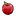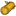## FANDOM

185 个页面

### 概要

• 一但你摧毁敌人城堡就会获得奖励宝箱，其内容取决于敌人的实力等级(Power Level)。
• 获胜的程度(星星数、摧毁建筑百分比)和奖励宝箱内容并无关系
• 将可在时代三开始获得文明旗帜与黄金。
• 将可在时代四开始获得石头。
• 即使没有研发奖励宝箱加成，也可以获得白金宝箱。

### 公式

• 假设N为敌人的实力等级。 (1 ≤ N ≤ 30).
• 在游戏一开始时，实力等级为1。如果你摧毁一个实力等级为一的敌人，你将会获得85和 85• 另一范例：若你摧毁一位时代八的敌人，且其实力等级为25，你的奖励宝箱加成也达到最大研究等级，则你将会在奖励宝箱中获得1525和/或 1525和/或 765和/或 26和/或 29.
• 白金宝箱奖赏是一般奖励宝箱的三倍！！

#### 宝箱= 25 + N * 60= 25 + N * 60= 15 + N * 30= N + 1= N + N * 奖励宝箱加成

#### 白金宝箱= (25 + N * 60) * 3= (25 + N * 60) * 3= (15 + N * 30) * 3= (N+1) * 3= (N + N * 奖励宝箱加成) * 3